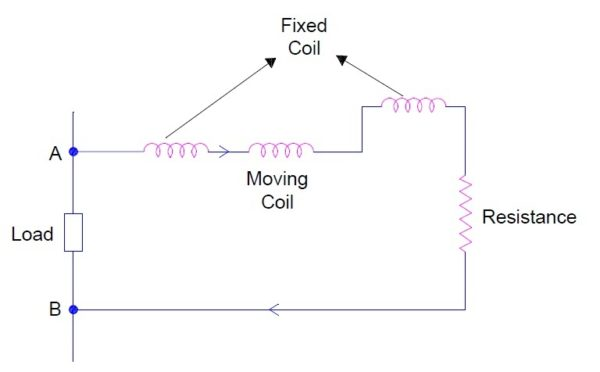# Torque Equation in Electrodynamometer Instruments

Electrodynamometer Instruments are widely used as an ammeter, voltmeter and wattmeter. This instrument can measure AC as well as DC quantities. This means that the torque in electrodynamometer instruments must have a finite value and unidirectional for AC and DC quantity unlike PMMC instruments. In this post we will be discussing the torque equation in such instruments.

### Torque Equation in Electrodynamometer Instruments

Electrodynamometer  instruments or Electrodynamic instruments have fixed coil divided into two sections and a moving coil. It is recommended to read before going to understand the torque equation. If you are already aware of constructional feature then you may proceed.

Let us assume that the current in fixed coil be I1 and that in moving coil be I2 as shown in figure below.

Also assume that,

L1 = Self inductance of fixed coil

L2 = Self inductance of moving coil

M = Mutual inductance between fixed and moving coils

Thus,

The flux linkage of fixed coil Ø1 = L1i1 + Mi2

The flux linkage of moving coil Ø2 = L2i2 + Mi1

The electrical energy input to the instrument,

= e1i1dt + e2i2dt

e1 = d Ø1/dt

and e2 = d Ø2/dt

Therefore energy input to the instrument

= i1d Ø1 + i2d Ø2

= i1d (L1i1 + Mi2) + i2d(L2i2 + Mi1)

= i1L1di1 + i12dL1 + i1i2dM + i1Mdi2 + i2L2di2 + i22dL2 + i1i2dM + i2Mdi1

Since L1 and L2 are constant, therefore dL1 = 0 and dL2 = 0

= i1L1di1 + i1i2dM + i1Mdi2 + i2L2di2 + i1i2dM + i2Mdi1 …………(1)

Some of the above input energy to electrodynamometer instruments are stored in the form of magnetic energy in the coil while rest is converted into mechanical energy of moving coil.

Thus we can write,

Energy Input = Mechanical Energy + Stored Energy

Mechanical Energy = Electrical Input – Stored Energy  …………(2)

Thus to find the mechanical energy, we need to find the change in stored energy in the magnetic field of the coil. Let us assume an infinitesimally small time dt for the sake of calculation of change in stored energy.

Change in stored energy

= d(1/2L1i12 + 1/2L2i22 + Mi1i2)

= i1L1di1+ i2L2di2+ i1Mdi2 + i2Mdi1+ i1i2dM+(i12/2)dL1 + (i22/2)dL2

But L1 and L2 are constant, therefore dL1 = 0 and dL2 = 0

= i1L1di1+ i2L2di2+ i1Mdi2 + i2Mdi1+ i1i2dM ……(3)

From equation (1), (2) and (3),

Mechanical Energy = i1i2dM

Let Td be the dflectiong torque and dƟ be the change in deflection, then mechanical energy

= Td

TddƟ = i1i2dM

⇒Td = i1i2dM/dƟ

The above equation gives the deflecting torque in electrodynamics or electrodynamometer instruments. It can be seen that deflecting torque depends upon the multiplication of instantaneous value of current and rate of change of mutual inductance between the fixed and moving coil.

Now we will consider two cases.

##### Case-1: When DC quantity is being measured.

Let I1 and I2 be the current in fixed and moving coil respectively. Therefore deflecting torque Td = I1I2dM/dƟ

But this deflecting torque is controlled by the spring. Spring provides the controlling torque. The controlling torque due to spring for a deflection of Ɵ

Tc = KƟ where K is spring constant.

At equilibrium the controlling torque and deflecting torques are equal, hence

Tc = Td

⇒KƟ = I1I2dM/dƟ

⇒Ɵ = (I1I2dM/dƟ)/K

##### Case-2: When AC quantity is being measured.

Let i1 and i2 are sinusoidal current having a phase displacement of Ø. Therefore we can write as

i1 = Im1Sinwt

i2 = Im2Sin(wt-Ø)

Thus the instantaneous deflecting torque is given as

Td = (Im1Sinwt)[ Im2Sin(wt-Ø)]dM/dƟ

The average torque for one time period of the currents are given by

Td = (I1I2CosØ)dM/dƟ

Where I1 = RMS Value of i1

I2 = RMS value of i2

From the above two cases, we can have following conclusions:

• For sinusoidal alternating current, the deflecting torque is determined by the product of RMS value of coil currents and the cosine of phase angle between them.
• When the instrument is used for AC, the instantaneous torque is proportional to i2. Thus the torque varies as the current varies but the direction of torque remains the same. Because of the inertia of the instrument, the needle does not follow the change in torque rather it takes a position where the average torque becomes equal to the controlling torque.

### Electrodynamometer Ammeters

Figure below shows an electrodynamometer ammeter.

It can be seen that, the fixed coil and moving coil are connected in series and hence carries the same current. As the current through the moving coil shall not exceed 100 mA, therefore moving coil is shunted by suitable resistance to increase the range of such ammeter.

As the currents flowing in fixed and moving coils are same therefore there will not be any phase angle between them. Therefore, Ø = 0

Deflecting Torque in Ammeter

Td = I2dM/dƟ

where I is the RMS current flowing in fixed and moving coil.

### Electrodynamometer Voltmeter

Figure below shows the connection arrangement for electrodynamometer voltmeter.In the figure above, voltmeter is connected between points A and B to measure the voltage drop across it. Note that a high value of resistance is connected in series with the coils. Why?

Since

Ø = 0

Therefore, Deflecting Torque in Ammeter

Td = I2dM/dƟ

where I is the RMS current flowing in fixed and moving coil. But I = VAB / Z where Z is the impedance of instrument circuit. Hence,

Td =( VAB/Z)2dM/dƟ

Electrodynamometer type voltmeters are the most accurate type of AC voltmeters. But the sensitivity of such instrument is low when compared from DC instruments.

### 3 thoughts on “Torque Equation in Electrodynamometer Instruments”

1.Rambabu kumar says:

Tanks sir

2.Moiz says:

It helps a lot thanks,Sir

1.admin says:

Thank you Moiz! Please share if you liked it.

This site uses Akismet to reduce spam. Learn how your comment data is processed.